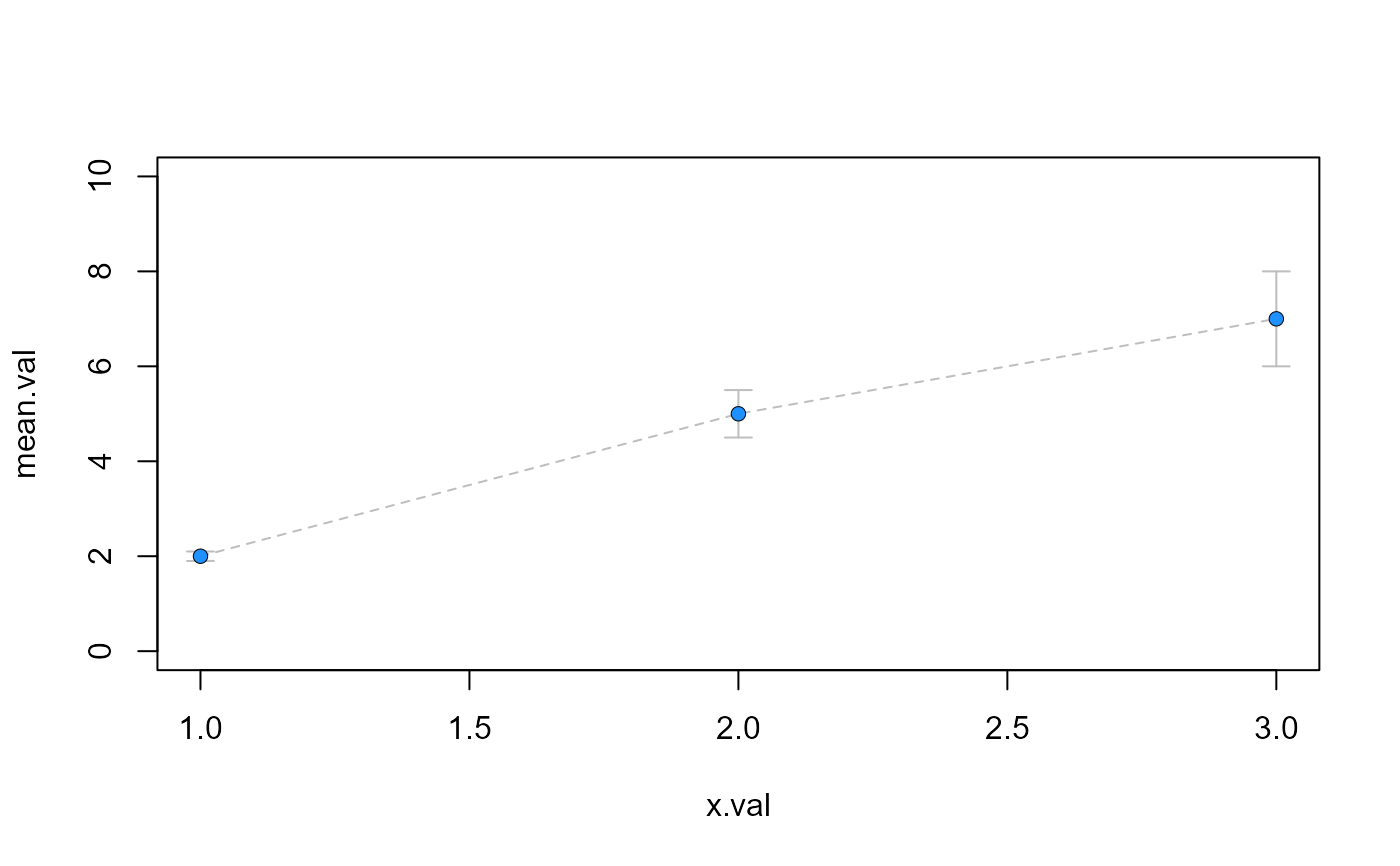Point with error bars

pt_line_error(
x,
y,
SE,
ln.lty,
ln.col,
ln.lwd,
pch,
bg,
cex = 1,
pt.lwd = 1,
pt.lty = 1,
er.lwd = 1,
pt.col = "black",
length = 0.07
)

## Arguments

x

x-value

y

y-value (or mean)

SE

standard error

ln.lty

line type

ln.col

color of lines

ln.lwd

width of lines

pch

plotting ‘character’, i.e., symbol to use. see points()

bg

background (fill) color for the open plot symbols given by pch = 21:25. see points()

cex

size of point

pt.lwd

line width of points

pt.lty

line type for points

er.lwd

error bar line width

pt.col

color of point

length

length of the edges of the error bar head (in inches).

## Value

draws lines in the form of error bars

## Note

see arrows(), errorbars() and pt_error() functions

## Examples

x.val=c(1,2,3)
mean.val=c(2,5,7)
se.val=c(0.1,0.5,1)
plot(mean.val~x.val,ylim=c(0,10),type="n")
pt_line_error(x.val,mean.val,se.val,2,"grey",1,21,"dodgerblue1")